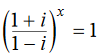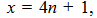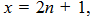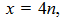Q

# I need help with If ,then

If,then

• Option 1)

where    is any positive integer

• Option 2)where    is any positive integer

• Option 3)where    is any positive integer

• Option 4)where    is any positive integer

119 Views
N

As we learnt in

Power of i in Complex Numbers -

- wherein

[For x = 4n]

Correct option is 4.

Option 1)

where    is any positive integer

This is an incorrect option.

Option 2)where    is any positive integer

This is an incorrect option.

Option 3)where    is any positive integer

This is an incorrect option.

Option 4)where    is any positive integer

This is the correct option.

Exams
Articles
Questions